International
Tables for
Crystallography
Volume B
Reciprocal space
Edited by U. Shmueli

International Tables for Crystallography (2006). Vol. B. ch. 1.2, p. 23   | 1 | 2 |

## Section 1.2.12.3. The one-particle potential (OPP) model

P. Coppensa*

aDepartment of Chemistry, Natural Sciences & Mathematics Complex, State University of New York at Buffalo, Buffalo, New York 14260-3000, USA
Correspondence e-mail: coppens@acsu.buffalo.edu

#### 1.2.12.3. The one-particle potential (OPP) model

| top | pdf |

When an atom is considered as an independent oscillator vibrating in a potential well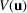, its distribution may be described by Boltzmann statistics.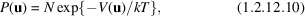with N, the normalization constant, defined by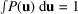. The classical expression (1.2.12.10)is valid in the high-temperature limit for which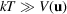.

Following Dawson (1967)and Willis (1969), the potential function may be expanded in terms of increasing order of products of the contravariant displacement coordinates: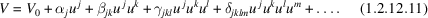The equilibrium condition gives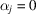. Substitution into (1.2.12.10)leads to an expression which may be simplified by the assumption that the leading term is the harmonic component represented by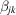: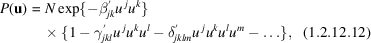in which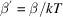etc. and the normalization factor N depends on the level of truncation.

The probability distribution is related to the spherical harmonic expansion. The ten products of the displacement parameters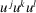, for example, are linear combinations of the seven octapoles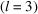and three dipoles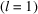(Coppens, 1980). The thermal probability distribution and the aspherical atom description can be separated only because the latter is essentially confined to the valence shell, while the former applies to all electrons which follow the nuclear motion in the atomic scattering model.

The Fourier transform of the OPP distribution, in a general coordinate system, is (Johnson, 1970a; Scheringer, 1985a)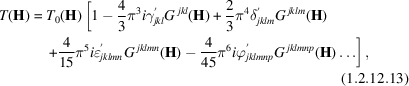where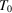is the harmonic temperature factor and G represents the Hermite polynomials in reciprocal space.

If the OPP temperature factor is expanded in the coordinate system which diagonalizes, simpler expressions are obtained in which the Hermite polynomials are replaced by products of the displacement coordinates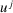(Dawson et al., 1967; Coppens, 1980; Tanaka & Marumo, 1983).

### ReferencesCoppens, P. (1980). Thermal smearing and chemical bonding. In Electron and magnetization densities in molecules and solids, edited by P. J. Becker, pp. 521–544. New York: Plenum.Google ScholarDawson, B. (1967). A general structure factor formalism for interpreting accurate X-ray and neutron diffraction data. Proc. R. Soc. London Ser. A, 248, 235–288.Google ScholarDawson, B., Hurley, A. C. & Maslen, V. W. (1967). Anharmonic vibration in fluorite-structures. Proc. R. Soc. London Ser. A, 298, 289–306.Google ScholarJohnson, C. K. (1970a). Series expansion models for thermal motion. ACA Program and Abstracts, 1970 Winter Meeting, Tulane University, p. 60.Google ScholarScheringer, C. (1985a). A general expression for the anharmonic temperature factor in the isolated-atom-potential approach. Acta Cryst. A41, 73–79.Google ScholarTanaka, K. & Marumo, F. (1983). Willis formalism of anharmonic temperature factors for a general potential and its application in the least-squares method. Acta Cryst. A39, 631–641.Google ScholarWillis, B. T. M. (1969). Lattice vibrations and the accurate determination of structure factors for the elastic scattering of X-rays and neutrons. Acta Cryst. A25, 277–300.Google Scholar